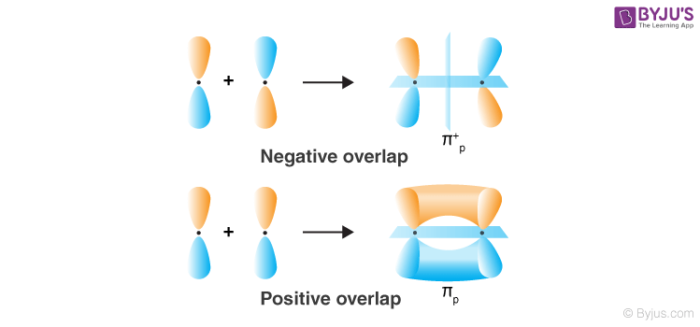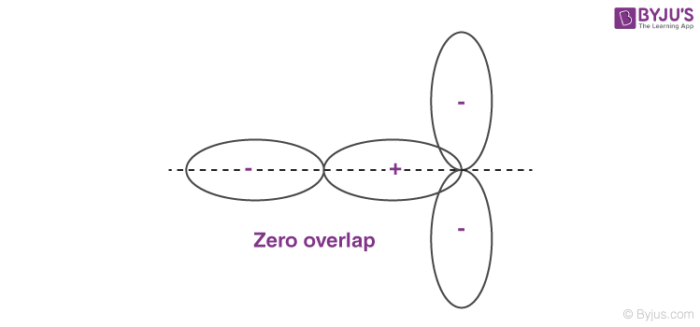# Orbital Overlap Concept

## What is Orbital Overlap?

The atoms combine by colliding with each other. But what does this mean on atomic level. This situation refers to the process in which the two atoms comes so close to each other that they penetrate each other’s orbital and form a new hybridized orbital where the bonding pair of electrons reside.  This hybridized orbital has lower energy than the atomic orbital and hence are stable. It is in the minimum energy state. This partial penetration of the orbital is known as orbital overlap.

The extent of overlap depends on the two participating atoms, their size and the valence electrons. In general, the greater the overlap, stronger is the bond formed between the two atoms. Thus, according to the orbital overlap concept, atoms combine by overlapping their orbital and thus forming a lower energy state where their valence electrons with opposite spin, pair up to form covalent bond.

The importance of orbital overlap was emphasized by Linus Pauling while explaining the molecular bond angles observed through experimentation and is the basis for the concept of orbital hybridization.

## Directional Properties of bond

The molecular bond angles were explained through the directional properties of the bond. The molecule of hydrogen is formed by overlap of 1s orbital in head on collision.

## Overlapping of Atomic Orbital

When two atoms come in contact with each other to form a bond, their overlap can be positive, negative or even zero depending upon the phase and sign of the two interacting orbital.

1. Positive Overlapping of Atomic Orbital – When the phase of two interacting orbitals is same, then the overlap is positive and in this case, the bond is formed. The phase of the two interacting orbital (+ or -) comes from the sign of orbital wave function and is not related to the charge in any sense.
1. Negative Overlapping of Atomic Orbital – When the phase of two interacting atomic orbital is opposite, then the overlap is negative and in this case, the bond is not formed.1. Zero Overlapping of Atomic Orbital – When the orientation of two interacting atomic orbital is such that there is no overlapping of the orbital, that is known as zero overlapping.## Frequently Asked Questions – FAQs

### Why do orbitals overlap?

Since they take up space, atomic orbitals overlap. An atomic orbital is a place of space where it is most possible that an electron will be detected. The two nuclei are attracted by the increased electron density and their repulsions are decreased. The outcome is that a covalent bond between H and Cl is formed.

### What is positive overlap?

Positive Atomic Orbital Overlap-If the phase of two interacting orbitals is the same, the overlap is positive and the bond is formed in this situation. The step of the two interacting orbitals (+ or-) emerges from the sign of the function of the orbital wave and is in no way related to the charge.

### What do you mean by zero overlap?

When two orbitals with separate phases intersect with each other, a negative phase overlap occurs. A zero overlap will result in orbitals not overlapping at all or not overlapping efficiently. A zero overlap between orbitals does not result in the forming of bonds.

### What are orbital phases?

Orbitals are essentially mathematical functions that define complex patterns of standing waves that can be graphed on a graph but do not have any physical reality. An orbital process is a direct result of electrons’ wave-like properties.

### What is meant by hybridization?

Orbital hybridization (or hybridization) in chemistry is the process of combining atomic orbitals into new hybrid orbitals (with different energies, sizes, etc., than atomic orbitals) suitable for pairing electrons in valence bond theory to form chemical bonds.

Take up a quiz on Optical overlap

#### 1 Comment

1. Vincent

Educative, I like it